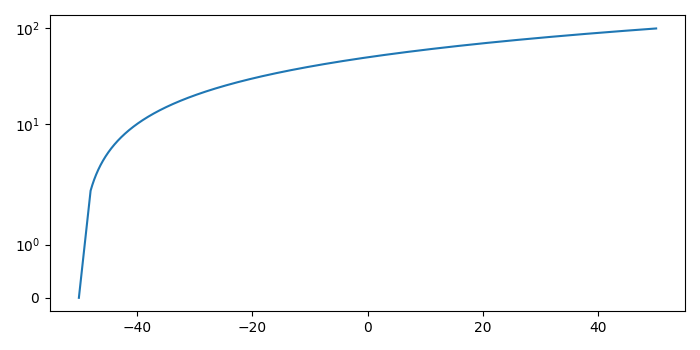# How to exponentially scale the Y axis with matplotlib?

To exponentially scale the Y-axis with matplotlib, we can take the following steps −

• Set the figure size and adjust the padding between and around the subplots.
• Inintialize a variable dt for steps.
• Create x and y data points using numpy.
• Plot the x and y data points using numpy.
• Set the exponential scale for the Y-axis, using plt.yscale('symlog').
• To display the figure, use show() method.

## Example

import numpy as np
import matplotlib.pyplot as plt

plt.rcParams["figure.figsize"] = [7.00, 3.50]
plt.rcParams["figure.autolayout"] = True

dt = 0.01

x = np.arange(-50.0, 50.0, dt)
y = np.arange(0, 100.0, dt)

plt.plot(x, y)
plt.yscale('symlog')

plt.show()

## Output

It will produce the following output